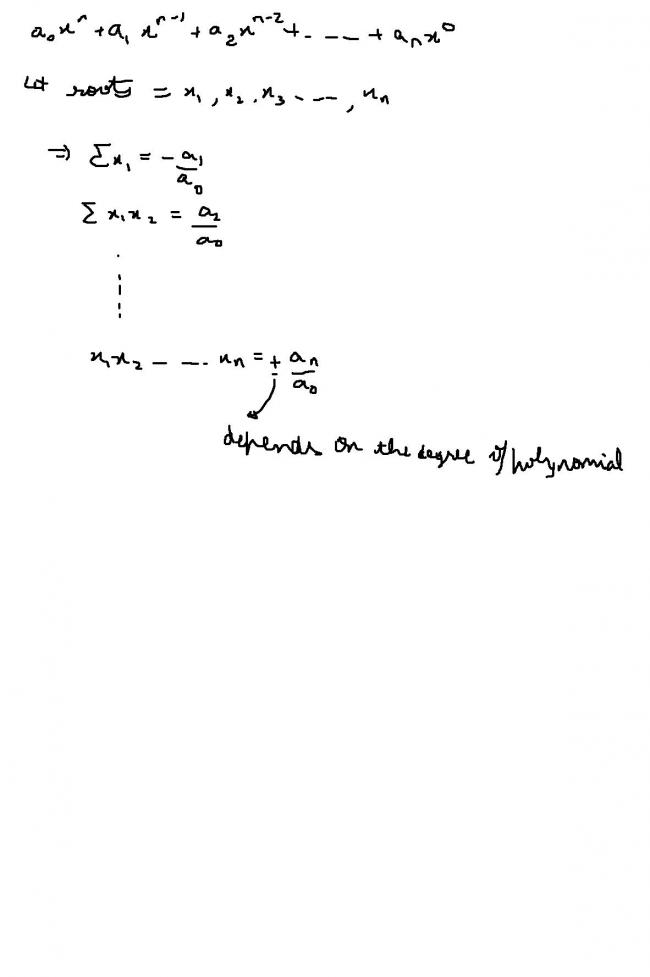# arithmetic

d arithmetic mean of d roots of d equatio n

4Cos3x -4Cos2x -Cos[315pie+x]=1

•virang1 Jhaveri ·

But eureka when i actually solved it i got a i = âˆš-1 in between how do i solve that

•2nÏ€=x is the answer as range of x is [0,315] so convert 315 into radian 315Ã—Ï€/Ï€â‰ˆ100Ï€ so x=0,2Ï€,4Ï€......100Ï€ and atithimetic mean comes out to be (100Ï€+0)/2=50Ï€

•eureka123 ·

the question is indeed wrong...........

right question is
the arithmetic mean of d roots of the equation

4Cos3x -4Cos2x -Cos(Ï€+x)=1 in interval (0,315)

solution:
proceeding as nishant sir did,we get cosx=1
=>cosx=2n.Ï€
Since 100Ï€<315<101Ï€
=>cosx=2Ï€,4Ï€,...........100Ï€

=>AM=2(Ï€+2Ï€+........50Ï€)/50
=>AM=2*50*51*Ï€/2*50
=>AM=51Ï€

•Lokesh Verma ·

do you mean that you got the answer 51 pi?

I think that is the answer

can you tell the other options

and also can you tell the source of this question?

•jb6098 swathi ·

idont understand wat ur saying bhaiyya d ans is given as 51âˆ© i got it can ne 1 say how it is plz

•Lokesh Verma ·

4Cos3x -4Cos2x -Cos[315pie+x]=1
=
4Cos3x -4Cos2x + Cosx-1=0

( 4 cos2x +1 ) (cos x - 1) =0

the first one is not possible...

so x=(2n)Ï€

among the options given to your brinda... you should realise that no other option will hold.. I think you must be solving a multiple choice question..

•brinda laxmi ·

•virang1 Jhaveri ·

ok so you take the other roots as nil and take only 1

•eureka123 ·

u wrote .....

a = i/2
=> cosx=i/2

writing this doesnt mean anything

i hope u unerstand.......

•kamalendu ghosh ·

it reduces to 4 cos3x -4cos2x+cosx-1=o

take cos x-1 common......

•eureka123 ·

for virang•eureka123 ·

i also missed to put 3 in place of 2...
corrected that...

•virang1 Jhaveri ·

Can you pls tell me the property i donot know that

•kamalendu ghosh ·

oops!! missed that

•eureka123 ·

when u had till here......4Cos3x - 4 Cos2x + Cosx = 1
then use the property.....
summation of roots=-(-4/4)=1
=> AM=1/3

•virang1 Jhaveri ·

4Cos3x - 4 Cos2x + Cosx = 1
let Cos x = a
4a3 - 4a2 + a = 1
4a3 - 4a2 + a - 1 = 0
(a - 1)(4a2 + 1) = 0
Therefore possible
a = 1
a = i/2
A.M = 1 + i /3
AM = 1/3 + i/3

•kamalendu ghosh ·

then (4cos2x+1)(cosx-1)=o....

so only cos x-1=0

so cos x=1

•